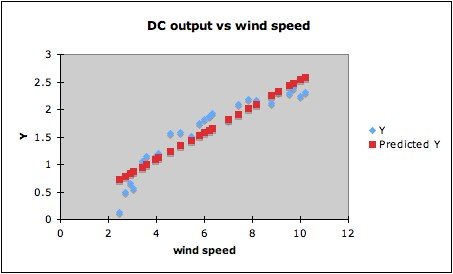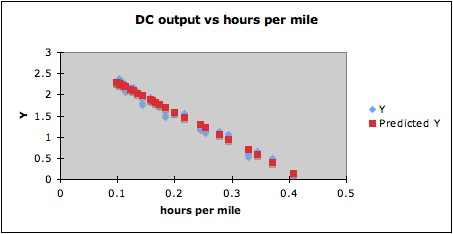# Overinterpreting High R2

1. Just what is considered high R2 varies from field to field. In many areas of the social and biological sciences, an R2 of about 0.50 or 0.60 is considered high. Yet Cook and Weisberg1 give an example of a simulated data set with 50 predictors and 100 observations, where the response was independent of all the predictors (so all regressors have coefficient zero in the true mean function), but R2 = 0.59.2

2. High R2 can also occur when overfitting. The R2 for the example of overfitting by a quartic curve was 1.00, since the curve went through all the points.

3. A regression model giving apparently high
R2 may not be as good a fit as might be obtained by a transformation. For example, fitting a linear regression to the following data (DC output of a windmill vs windspeed) will give R2 = 0.87. (Data in blue, regression line in red.)This for some purposes might be a good enough fit. However, since the data indicate a clear curved trend, it is likely that a better fit can be found by a suitable transformation.  Since the predictor windspeed is a rate (miles per hour), one possibility is that the reciprocal (hours per mile) might be a natural choice of transformation. Trying this gives R2  = 0.98, and the plot below shows that indeed a linear fit for the transformed data makes more sense than for the untransformed data.Notes:
1. R.D. Cook and S. Weisberg (1999),
Applied Regression Including Computing and Graphics, Wiley, p. 281.
2. The p-value of the F-statistic for significance of the overall regression was 0.13, however. But six of the terms were individually significant at the .05 level.

Last updated June 13, 2014